Next: Needham-Schroeder key distribution protocol Up: Proofs of Exemplary Protocols Previous: Proofs of Exemplary Protocols

## Station-to-Station Protocol

This section presents the proofs of the first half of the station to station (STS) protocol [DvOW92]. It shows that A creates a fresh, good key (K) for use with B. The second half, showing the same for B would be quite similar.

The interesting thing about this protocol is that A produces key (K) twice. The first time A uses a function operation which allows A to see K and to believe K is fresh. The second time A uses the create key operation which proves K is a good key.

The STS protocol:

1. AB : R2. BA : R,(B,K,[B,K]),{[R,R]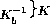3. AB : R,(A,K,[A,K]),{[R,R]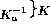Expanded protocol in our notation, from A's point of view:

STS1
A: RSTS2A : R,(B,K,[B,K]),{[R,R]STS3
A : F(R,x) = K = (R){A creates fresh K, but doesn't believe its good yet.}
STS4
A : decrypt [B,K]
STS5
A : proves A believes PK(B,K)
STS6
A : decrypt {[R,R]{A uses K}
STS7
A : decrypt [R,R]
STS8
A : proves A believes PK(B,R)
STS9
A : CreateKey K = F(PK(B,R),PK(A,R)) {A recreates K, believes its good}

We use the following as A's postconditions. We understand that this is not a complete set, but is sufficient to demonstrate our technique. A full set of post conditions would consist of G1-5 as defined in [SvO96].

• A believes (A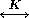B) Discharged in step 9
• A sees [R, R] Discharged in step 7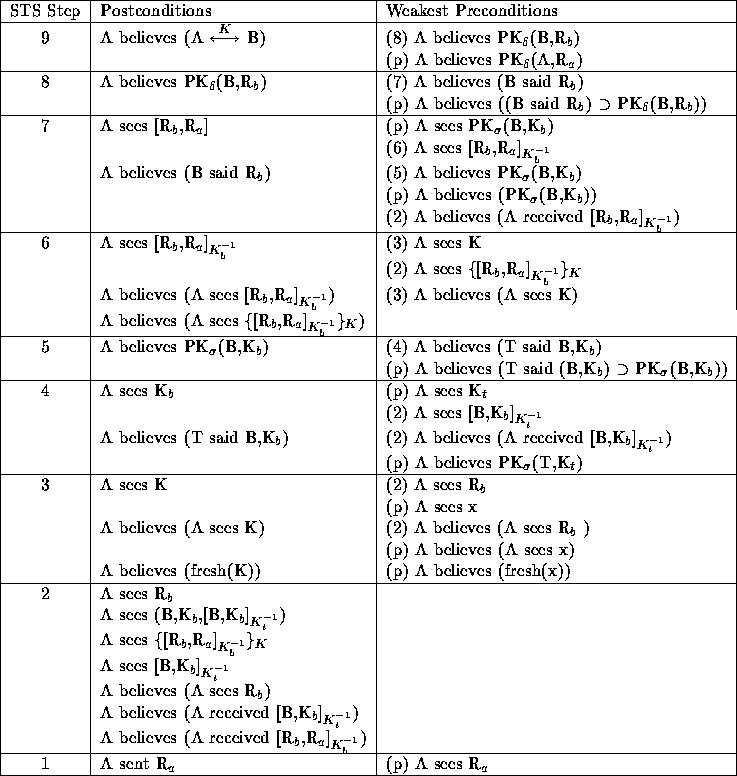Table 13: Proof of A's postconditions for the STS protocol.

The generated premises, from A's point of view are:

• A sees x,R,K,PK(B,K)
• Also A believes it sees each of the above
• A believes (fresh(x))
• A believes PK(A,R)
• A believes (T said (B,K)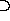PK(B,K)
• A believes ((B said R)PK(B,R))

These premises are generated through direct application of the wp rules given in the previous section. Since we are generating preconditions from postconditions, we work backwards from the last step of the protocol to the first. In Table 13 we have labeled the generated preconditions with either the step in which they are discharged, or with a (p) to denote that they should be considered a premise of the protocol. For example in step 9 we apply the CreateKey rule from Table 5 to the valid key creation postcondition, the generated preconditions are discharged in step 8 and as a premise. In step 8 we apply the proof wps from Table 6 to the wp generated in step 9, the generated preconditions are discharged in step 8 and as a premise.We continue with this through the remainder of the protocol.Next: Needham-Schroeder key distribution protocol Up: Proofs of Exemplary Protocols Previous: Proofs of Exemplary Protocols

Jim Alves-Foss
Fri Aug 1 16:00:31 PDT 1997